## A Systematic Algorithm for the Synthesis of Multiplierless Lattice Wave Digital Filters

J. Yli-Kaakinen and T. Saramäki, "A Systematic Algorithm for the Synthesis of Multiplierless Lattice Wave Digital Filters," invited book chapter in Digital Filters, Fausto Pedro García Márquez (Ed.), ISBN: 978-953-307-190-9, Intech, pp. 257–289, Apr. 2011

Full text available as: PDF (1.2 MB) – Requires Adobe Acrobat Reader or other PDF viewer.

Electronic version of an article published in http://www.intechopen.com/articles/show/title/a-systematic-algorithm-for-the-synthesis-of-multiplierless-lattice-wave-digital-filters

### Abstract

Among the best structures for implementing recursive digital filters are lattice wave digital (LWD) filters (parallel connections of all-pass filters). They are characterized by many attractive properties, such as a reasonably low coefficient sensitivity, a low roundoff noise level, and the absence of parasitic oscillations. This book chapter describes an efficient algorithm for the design of multiplierless LWD filters in the following three cases. In the first case, the overall filter is constructed as a cascade of low-order LWD filters. As a consequence, the number of bits required for both the data and coefficient representations are significantly reduced compared with the conventional direct-form LWD filter. In the second case, approximately linear-phase LWD filters are constructed as a single block because it has been observed that in this case the use of a cascade of several filter blocks does not provide any benefits over the direct-form LWD filter design. The third case concentrates on the design of special recursive single-stage and multistage Nth-band decimators and interpolators providing the sampling rate conversion by the factor of N. For this filter class, the decimation and interpolation filter in the single-stage design (the kth decimation and interpolation filter in the multistage design, where N is factorizable as a product of K integers as N=N1N2NK) is characterized by the fact that it can be decomposed into parallel connection of N (Nk) polyphase components that are obtainable from cascades of first-order all-pass filters by substituting for each unit delay N (Nk) unit delays.

The coefficient optimization is performed using the following three steps. First, an initial infinite-precision filter is designed such that it exceeds the given criteria in order to provide some tolerance for coefficient quantization. Second, a nonlinear optimization algorithm is used for determining a parameter space of the infinite-precision coefficients including the feasible space where the filter meets the given criteria. The third step involves finding the filter parameters in this space so that the resulting filter meets the given criteria with the simplest coefficient representation forms. The proposed algorithm guarantees that the optimum finite-precision solution can be found for the multiplierless coefficient representation forms. Filters of this kind are very attractive in very large-scale integration (VLSI) implementations because the realization of these filters does not require the use of one or many very costly general multiplier elements. Several examples are included to illustrate the benefits of the proposed synthesis scheme as well as the resulting filters.

### Errata

A list of errata can be found here: Intech_Errata.pdf (59 KB)

### BibTeX

``` @InCollection{ylikaaki11a,   author = {J. Yli-Kaakinen and T. Saram{\"a}ki},   title = {A Systematic Algorithm for the Synthesis of Multiplierless Lattice Wave Digital           Filters},   booktitle = {Digital Filters},   pages = {257--289},   publisher = {Intech},   year = 2011,   editor = {F. P. G. M{\'a}rquez},   chapter = 11,   month = {Apr.} } ```

### Examples

#### Example 1

A c-language implementation of the multiplierless lattice wave digital filter: cascadeFilter.c. An input file of the filter: sines_long.bin.

``` % Matlab/octave code for generating the input file fin = [1.0e3; 23.25e3; 11.5e3; 5.75e3]; % Input frequencies fs = 48e3; % Sampling frequency nSamples = 2^15; t = (0:nSamples-1)/fs; x = sum(cos(2*pi*fin(:)*t), 1)/numel(fin); % x ∈ [–1, 1] xint = round(x*(2^31-1)); % xint ∈ [–2147483647, 2147483647] fid = fopen('sines_long.bin', 'w'); fwrite(fid, xint, 'integer*4'); fclose(fid); ```

_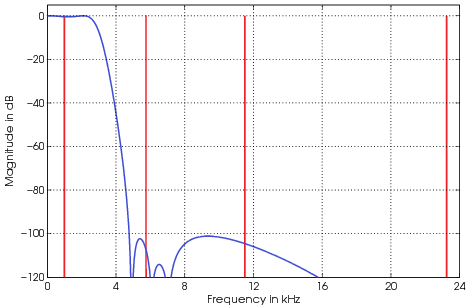Fig: Magnitude responses for the optimized finite-precision multiplierless lattice wave digital filter in Example 1 as well the magnitude spectrum of the input signal consisting of four sinusoidals at 1 kHz, 5.75 kHz, 11.5 kHz, and 23.25 kHz.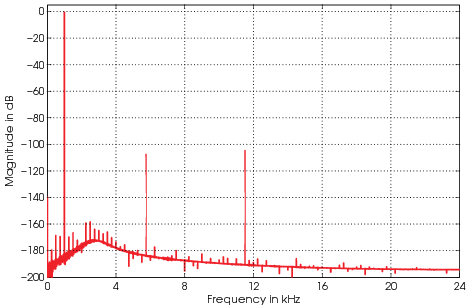Fig: Magnitude spectrum of the output signal.

#### Example 3

A c-language implementation of the multiplierless 8-to-1 decimator: decimator8to1.c. An input file of the decimator: sines.bin.

``` % Matlab/octave code for generating the input file fin = [1.0e3; 23.25e3; 11.5e3; 5.75e3]; % Input frequencies fs = 48e3; % Sampling frequency nSamples = 2^15; t = (0:nSamples-1)/fs; x = sum(cos(2*pi*fin(:)*t), 1)/numel(fin); % x ∈ [–1, 1] xint = round(x*(2^15-1)); % xint ∈ [–32767, 32767] fid = fopen('sines.bin', 'w'); fwrite(fid, xint, 'integer*2'); fclose(fid); ```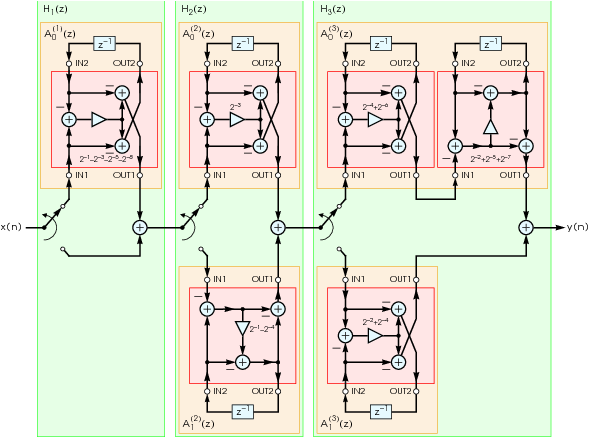Fig: An efficient implementation for the optimized finite-precision three-stage eighth-band decimator in Example 3.Fig: Magnitude responses for the optimized finite-precision three-stage eighth-band decimator in Example 3. The solid line gives the magnitude response for the single-stage equivalent H1(z)H2(z2)H3(z4), whereas the dotted, dot-dashed, and dashed lines give the responses for H1(z), H2(z2), and H3(z4), respectively.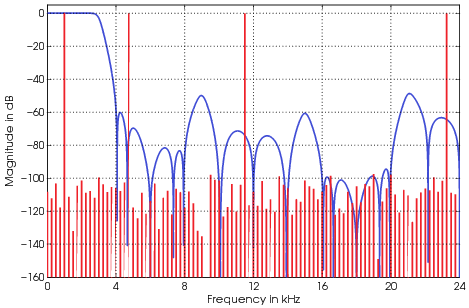Fig: Magnitude responses for the optimized finite-precision three-stage eighth-band decimator in Example 3 as well the magnitude spectrum of the input signal consisting of four sinusoidals at 1 kHz, 4.75 kHz, 11.5 kHz, and 23.25 kHz.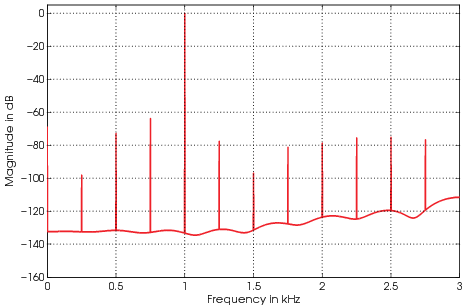Fig: Magnitude spectrum of the output signal after decimation by eighth. As can be seen from this figure the minimum attenuation of the aliasing terms is in this case roughly 64 dB.